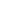| lesson material | material summary | questions and answers | definitions | types and examples | other information | materi pelajaran | ringkasan materi | pertanyaan dan jawaban | definisi | jenis-jenis dan contoh-contoh | informasi lainnya |

# Learning Javascript # 6: Six Types of Operators You Must Know in Javascript

After you learn about Variables and Data Types in Javascript , the next material that must be learned is about operators.
Operators are the basic things that must be understood in programming.
Because we will use it a lot to do various kinds of operations in the program.
What is an operator?
What operators are there in Javascript?
and how do you use it?
Let's be students ...

Suppose we have two variables like this:
``````var a = 3;
var b = 4;
``````
How do you grow variables `a`and `b`?
The answer: use a plus sign ( `+`).
``````var c = a + b;
``````
The sum will be stored in the variable `c`.
A plus sign `+`is an operator.
So…

## What is an operator?

An operator is a symbol used to perform operations on a value and variable.
Operators in programming are divided into 6 types:
1. Arithmetic operator;
2. Assignment Operator;
3. Opeartor relation or comparison;
4. Logic Operator;
5. Bitwise operator;
6. Ternary operators;
Mandatory operators exist in every programming language. You must understand the 6 types of operators above.
Let's discuss them one by one…

## 1. Opeartor Arithmetic in Javascript

Arithmetic operators are operators to carry out arithmetic operations such as addition, subtraction, division, multiplication, etc.
The arithmetic operator consists of:
Operator NameSymbol
Reduction-
Multiplication*
Appointment**
Division/
Remaining Share%
To do multiplication operations, we use an asterisk symbol `*`.
Don't use it `x`, because symbols are `x` not included in the operator in programming.
Then for appointment we use double asterisks `**`.
For division, we use slash symbols `/`.
Let's try ...
Example:
``````var a = 5;
var b = 3;

// menggunakan operator penjumlahan
var c = a + b;
console.log(c);
``````
The result: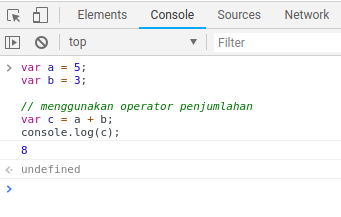Try also for other operators:
``````<!DOCTYPE html>
<html lang="en">

<title>Operator Aritmatika</title>

<body>

<script>
var a = 15;
var b = 4;
var c = 0;

// pengurangan
c = a - b;
document.write(`\${a} - \${b} = \${c}<br/>`);

// Perkalian
c = a * b;
document.write(`\${a} * \${b} = \${c}<br/>`);

// pemangkatan
c = a ** b;
document.write(`\${a} ** \${b} = \${c}<br/>`);

// Pembagian
c = a / b;
document.write(`\${a} / \${b} = \${c}<br/>`);

// Modulo
c = a % b;
document.write(`\${a} % \${b} = \${c}<br/>`);
</script>
</body>

</html>
``````
The result: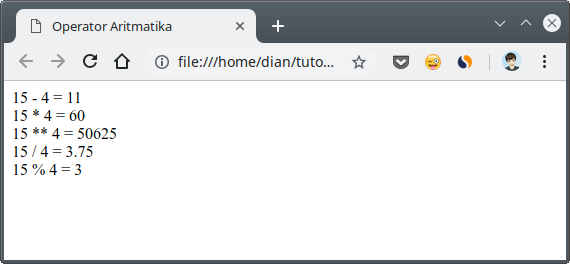Try matching the modulo ( `%`) operator and addition ( `+`operator .
The modulo operator is an operator to calculate the remainder for.
For example `3`divided `2`, then the rest is `1`.
``````3 % 2 = 1
``````

### Opertor Merge Text

Do not be Wrong.
In Javascript, if we are going to perform operations on string data types or text using addition ( `+`), then what will happen is merging ; Not summation.
Example:
``````var a = "10" + "2";
``````
Then the results will be:
``````102
``````
Why not `12`?
Because the two numbers are strings — notice, they are enclosed in quotation marks.
For other operations, please try it through the console .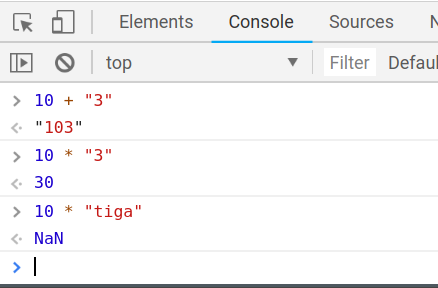## 2. Opeartor Assignment in Javascript

Assignment operators are operators that are used to assign assignments to variables. Usually used to fill variables.
Example:
``````var a = 19;
``````
`a`We assign assignment variables to store values `19`.
The assignment operator consists of:
Operator NameSombol
Charging Value=
Charging and Reduction- =
Filling and Multiplication* =
Filling and Lifting** =
Filling and Distribution/ =
Charging and Remaining for% =
Assignment operators are the same as arithmetic operators. It is also used for arithmetic operations.
Example:
``````var jumlahView = 12;

// menggunakan operator penugasan penjumlahan
// untuk menambah nilai
jumlahView += 1;
``````
The result:
The variable `jumlahView`will increase by one.
The purpose of `jumlahView += 1`is like this:
``````jumlahView = jumlahView + 1;
``````
Fill in the variable `jumlahView`with the sum of the `jumlahView`previous values with `1`.
Especially for assignment operators which are summed and subtracted by one, can be abbreviated with `++`and `--`for deduction.
Example:
``````var a = 2;
a++;
``````
Then the value of the variable `a`will be `3`.
Then the question:
What is the difference with an assignment operator with an arithmetic operator?
Arithmetic operators only carry out arithmetic operations, while assignment operators ... they carry out arithmetic operations and also fill.
The following are examples of assignment operators:
``````<!DOCTYPE html>
<html lang="en">

<title>Operator Penugasan</title>

<body>

<script>
document.write("Mula-mula nilai score...<br>");
// pengisian nilai
var score = 100;
document.write("score = "+ score + "<br/>");

// pengisian dan menjumlahan dengan 5
score += 5;
document.write("score = "+ score + "<br/>");

// pengisian dan pengurangan dengan 2
score -= 2;
document.write("score = "+ score + "<br/>");

// pengisian dan perkalian dengan 2
score *= 2;
document.write("score = "+ score + "<br/>");

// pengisian dan pembagian dengan 4
score /= 4;
document.write("score = "+ score + "<br/>");

// pengisian dan pemangkatan dengan 2
score **= 2;
document.write("score = "+ score + "<br/>");

// pengisian dan modulo dengan 3;
score %= 3;
document.write("score = "+ score + "<br/>");
</script>
</body>

</html>
``````
The result: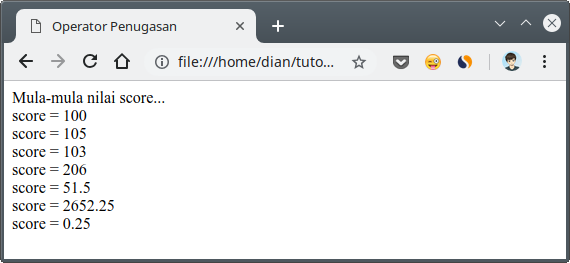## 3. Opeartor Comparison in Javascript

A relation or comparison operator is an operator used to compare two values.
The comparison operator will produce a boolean value `true`and `false`.
The comparison operator consists of:
Operator NameSymbol
Greater than>
Smaller<
Together with== or ===
Not equal to! = or! ==
Bigger Same with> =
Smaller Same as<=
Example:
``````<!DOCTYPE html>
<html lang="en">

<title>Operator Perbandingan</title>

<body>

<script>
var aku = 20;
var kamu = 19;

// sama dengan
var hasil = aku == kamu;
document.write(`\${aku} == \${kamu} = \${hasil}<br/>`);

// lebih besar
var hasil = aku > kamu;
document.write(`\${aku} > \${kamu} = \${hasil}<br/>`);

var hasil = aku >= kamu;
document.write(`\${aku} >= \${kamu} = \${hasil}<br/>`);

// lebih kecil
var hasil = aku < kamu;
document.write(`\${aku} < \${kamu} = \${hasil}<br/>`);

var hasil = aku <= kamu;
document.write(`\${aku} <= \${kamu} = \${hasil}<br/>`);

var hasil = aku != kamu;
document.write(`\${aku} != \${kamu} = \${hasil}<br/>`);
</script>
</body>

</html>
``````
The result: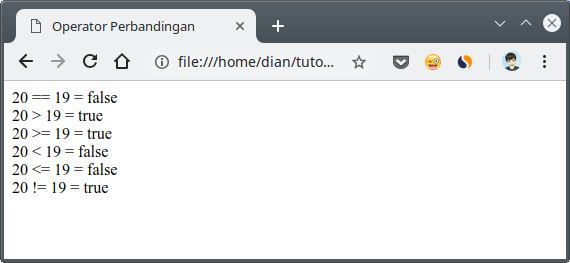The question:
What is the difference `==`(two symbols equal to) with `===`(three symbols equal to)?
Comparisons using symbols `==`will only compare values. Whereas those who use `===`will compare with the data type too.
Example:
``````// ini akan bernilai true
var a = "4" == 4; //-> true

// sedangkan ini akan bernilai false
var b = "4" === 4; //-> false
``````
Why is the value `b`valuable `false`?
Because `"4"`(string) and `4`(integer). The data type is different.

## 4. Opeartor Logic in Javascript

Logical operators are used to carry out operations on two values `boolean`.
This operator consists of:
Operator NameSymbol
Logic AND&&
Logic OR||
Negation / reverse!
Example:
``````<!DOCTYPE html>
<html lang="en">

<title>Operator Logika</title>

<body>

<script>
var aku = 20;
var kamu = 19;

var benar = aku > kamu;
var salah = aku < kamu;

// operator && (and)
var hasil = benar && salah;
document.write(`\${benar} && \${salah} = \${hasil}<br/>`);

// operator || (or)
var hasil = benar || salah;
document.write(`\${benar} || \${salah} = \${hasil}<br/>`);

// operator ! (not)
var hasil = !benar
document.write(`!\${benar} = \${hasil}<br/>`);

</script>
</body>

</html>
``````
The result: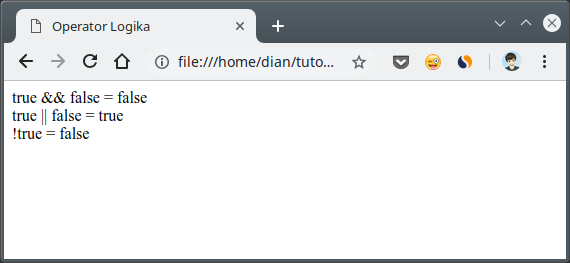## 5. Opeartor Bitwise in Javascript

The bitwise operator is an operator that is used for bit based operations.
This operator consists of:
NameSymbol in Java
AND&
OR|
XOR^
Negation / reverse~
Left Shift<<
Right Shift>>
Left Shift (unsigned)<<<
Right Shift (unsigned)>>>
This operator applies for the data type `int``long``short``char`, and `byte`.
This operator will calculate from bit-to-bit.
For example, we have variables `a = 60`and `b = 13`.
When made in binary form, it will be like this:
``````a = 00111100
b = 00001101
``````
(note the binary numbers, numbers `0`and `1`)
Then, do the bitwise operation
Operation AND
``````a     = 00111100
b     = 00001101
a & b = 00001100
``````
OR operation
``````a     = 00111100
b     = 00001101
a | b = 00111101
``````
XOR operation
``````a     = 00111100
b     = 00001101
a ^ b = 00110001
``````
NOT Opportunity
``````a   = 00111100
~a  = 11000011
``````
The concept is almost the same as Opeartor Logic. The difference is, Bitwise is used for binary.
For more details…
Let's look at an example:
``````<!DOCTYPE html>
<html lang="en">

<title>Operator Bitwise</title>

<body>

<script>
var x = 4;
var y = 3;

// operator bitwise and
var hasil = x & y;
document.write(`\${x} & \${y} = \${hasil}<br/>`);

// operator bitwise or
var hasil = x | y;
document.write(`\${x} | \${y} = \${hasil}<br/>`);

// operator bitwise xor
var hasil = x ^ y;
document.write(`\${x} ^ \${y} = \${hasil}<br/>`);

// operator negasi
var hasil = ~x;
document.write(`~\${x} = \${hasil}<br/>`);

// operator bitwise right shift >>
var hasil = x >> y;
document.write(`\${x} >> \${y} = \${hasil}<br/>`);

// operator bitwise right shift <<
var hasil = x << y;
document.write(`\${x} << \${y} = \${hasil}<br/>`);

// operator bitwise right shift (unsigned) >>>
var hasil = x >>> y;
document.write(`\${x} >>> \${y} = \${hasil}<br/>`);

</script>
</body>

</html>
``````
The result: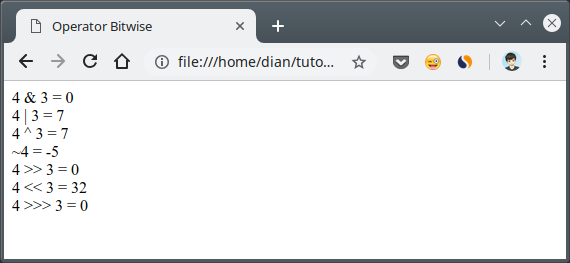## 6. Opeartor Ternary in Javascript

Finally there are Ternary operators ...
The ternary operator is an operator consisting of three parts.
Previous operators are only two parts, namely: the left and right parts. This is called a binary operator.
While trinary operators have parts left, center, and right.
``````bagian kiri <operator> bagian tengah <operator> bagian kanan
``````
The ternary operator in JavaScript is usually used to create an if / else branch .
The ternary opertor symbol consists of a question mark and a colon ( `?:`).
Form it like this:
``````<kodisi> ? "benar" : "salah"
``````
Pay attention! `<kondisi>`we can fill with expressions that produce values `true`and `false`.
If the condition is valuable `true`, then `"benar"`what will be chosen and vice versa - if - `false`then `"salah"`what will be chosen.
This operator is unique, like making questions.In the example above, "You like me" is the question or condition that will be examined.
If the answer is correct, then `iya`Otherwise it will `tidak`.
For more details, let's try an example.
``````<!DOCTYPE html>
<html lang="en">

<title>Operator Ternary</title>

<body>

<script>
var pertanyaan = confirm("Apakah kamu berumur diatas 18 tahun?")

var hasil = pertanyaan ? "Selamat datang" : "Kamu tidak boleh di sini";
document.write(hasil);
</script>
</body>

</html>
``````
The result:Sumber: https://www.petanikode.com/javascript-operator/
##### 0 Komentar untuk "Learning Javascript # 6: Six Types of Operators You Must Know in Javascript"

Silahkan berkomentar sesuai artikel

Template By Kunci Dunia